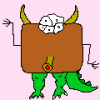https://leetcode-cn.com/problems/longest-substring-without-repeating-characters/

题目

``````输入: "abcabcbb"

``````

``````输入: "bbbbb"

``````

``````输入: "pwwkew"

请注意，你的答案必须是 子串 的长度，"pwke" 是一个子序列，不是子串。
``````

• 无重复字符
• 最长子串

解题

第一次提交

``````class Solution {
public int lengthOfLongestSubstring(String s) {
TreeSet<Integer> tempRes = new TreeSet<>();
Stack<Character> result = new Stack<>();
char[] inputs = s.toCharArray();
for (int i = 0; i < inputs.length; i++) {
char current = inputs[i];
if (result.contains(current)) {
result.clear();
}
result.push(current);
}
tempRes.comparator();
return tempRes.last();
}
}
``````

i堆栈长度
push('d')
0{d}1
push('v')
1{d, v}2
push('d')出现重复字符串，保存当前长度2，清空堆栈
push('d')
2{d}1
push('f')
3{d,f}2

第二次提交

``````class Solution {
public int lengthOfLongestSubstring(String s) {
TreeSet<Integer> tempRes = new TreeSet<>();
char[] inputs = s.toCharArray();
for (int i = 0; i < inputs.length; i++) {
char current = inputs[i];
if (result.contains(current)) {
result.remove();
i--;
} else {
}
}
tempRes.comparator();
return tempRes.last();
}
}
``````

i队列长度
0{d}1
1{d, v}2
2{v, d}2
3{v,d,f}3

第三次提交

``````class Solution {
public int lengthOfLongestSubstring(String s) {
if (s.isEmpty()) {
return 0;
}
int[] position = new int;
for (int i = 0; i < position.length; i++) {
position[i] = -1;
}
int max = 0;
int cur = 0;
for (int i = 0; i < s.length(); i++) {
int pos = s.charAt(i);
if (position[pos] < 0) {
cur += 1;
} else {
int gap = i - position[pos];
if (cur >= gap) {
cur = gap;
} else {
cur += 1;
}
}
position[pos] = i;
max = Math.max(max, cur);
}
return max;
}
}
``````

"pwwkew" 为例子，执行步骤：

icurmaxgap
011
122
2121
322
433
5333

总结

• 合适的数据结构事半功倍
• 针对字符串操作的题目，边界和特殊情况一定要多考虑。4评论
•Musir

贵州人？

•onlyloveyd 博主

@Musir 武汉人在在贵阳定居

•狮子陪我

楼主在吗，想问你下你以前写的flutter的问题，能给下联系方式吗，求解

•onlyloveyd 博主

@狮子陪我 QQ: 457420045